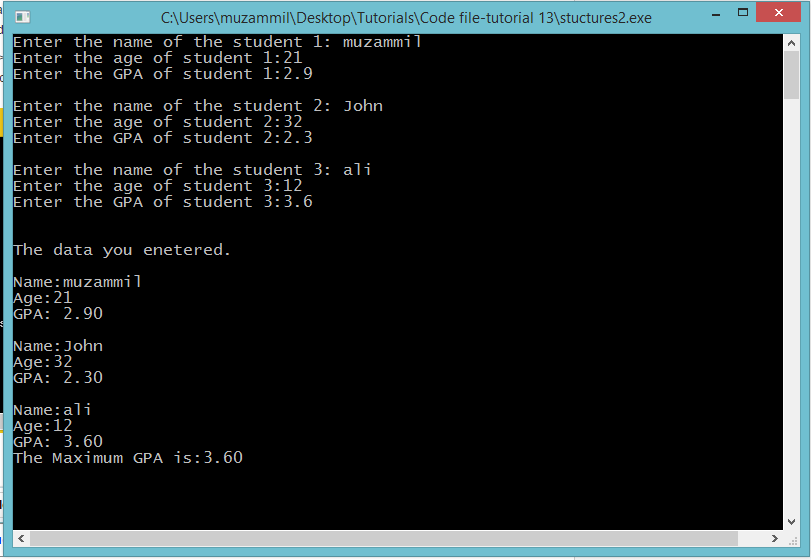# Structures in C

## Structures in C

In this part you will learn: 1. C syntax 2. Structure variable 3. Structure declaration 4. Structure accessing 5. Showing output

### What is a Structure?

Structure is a user-defined data type in C which allows you to combine different data types to store a particular type of record. Structure helps to construct a complex data type in a more meaningful way. Structure helps when you have to store a collection of data of many similar things or people etc. It is somewhat similar to an array. The difference is that an array is used to store a collection of similar type of data while structure can store collection of any type. Structure are best used to represent a record. If you want to store a record of hundred student you will need to define hundred variables to store the name and similarly hundred variables for each type of data you need to enter in the record. This is where structures come in handy. In this program we will make a structure of student that will store the name of the student, the age of the student and the gpa. Student Structure Basic Step: Open Dev C++ then File > new > source file and start writing the code below.
`#include<stdio.h>#include<conio.h>`
These two are the most common and basic header files. The first one stdio.h is used to grant us most of the basic functions of C like Input and Output functions. The second one conio.h provides us functions like getch() which is used to pause our screen until we press a button.
`struct Student{	char name;	int age;	float gpa;};`
We define structure before the main function. We write the struct keyword and the name of the structure. You can give any name to the structure except any keyword. Then in body of the structure we declare a char array of size 50 for storing the name the student. The and integer for storing the age and a float variable for the gpa. We close the brackets and place a semi colon at the end. Now when ever we will make a variable of student type all these three variables will be created in the memory.
`int main(){ 		Student s; 	int i,j; 	for(i=0; i<3;i++)	{		printf("Enter the name of the student %d: ",i+1);		scanf(" %s",&s[i].name); 		printf("Enter the age of student %d:",i+1);		scanf("%d",&s[i].age); 		printf("Enter the GPA of student %d:",i+1);		scanf("%f",&s[i].gpa); 		printf("\n"); 	}  	float maxgpa;	int l; 	for(l=0;l<3;l++)	{		if(l==0)		maxgpa=s[l].gpa;		else if(maxgpa<s[l].gpa)		{			maxgpa=s[l].gpa;		}	} `
Now we started the main function. In the main function we created a student array of size 3. That means we created three students. Then we started a for loop and took input in the array. Now every index of the array contains three types of data i.e. name of the student, age of the student and the gpa of the student. When taking input in the name of the student we just placed %s as format specifier and accessed the name variable with the ‘.’ Operator with the index. In this way we access any element of the structure. In the same way we took the input the other variables and the other students. After taking input in all the students we went for calculating the maximum gpa of the student which we did by using a for loop and comparing the gpas of students with eachother and stored the maximum gpa in another variable.
`printf("\nThe data you enetered.\n"); 	int m;	for(m=0; m<3;m++)	{		printf("\nName:%s",s[m].name); 		printf("\nAge:%d",s[m].age); 		printf("\nGPA: %0.2f",s[m].gpa);		printf("\n");  	} 	printf("The Maximum GPA is:%0.2f",maxgpa);  	getch();}`
After this we again implemented a for loop to display all the record of the students one by one. Then at last we displayed the maximum gpa of the students. And at the end we paused the program. Execute > compile then Execute > run Output